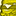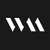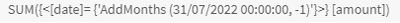# App Development

Announcements
Qlik Cloud Maintenance is scheduled between March 27-30. Visit Qlik Cloud Status page for more details.
cancel
Showing results for
Did you mean:Contributor II

## Sum expression with AddMonths to a variable

I created a variable vLatestDate which is derived from the max month of the [date] columnvLatestDate:

``Max([date])``

The following code gives me the sum of [amount] where [date] equals to vLatestDate:

``SUM({<[date]= {'\$(vLatestDate)'}>} [amount])``

I am now trying to create a column that is a sum expression based on the [date] column matching the vLatestDate variable minus one month.

I have tried the following code but it does not work:

``SUM({<[date]= {'\$(AddMonths(vLatestDate, -1))'}>} [amount]) ``Labels (1)
• ### General Question

1 Solution

Accepted SolutionsPartner - Contributor III

The issue appears to be that you're not telling Qlik that to actually calculate the AddMonths-function, it's being interpreted literally instead of executing.

Try the following measure:

``SUM({<[date]= {'\$(=AddMonths(\$(vLatestDate), -1))'}>} [amount]) ``

Note the addition of "="-character to the start of the dollar expansion, to tell Qlik to calculate the dollar expansion and not simply do a string replace. Sometimes the end result of your date adjustment might not meet the format of your target field, but in this case it should be fine. But if you wanted to for example do a MonthYear-field selection based on your vLatestDate, you'd have to add a formatting function after the AddMonths, such as MonthName-function or whichever format the target field is in.

4 RepliesMaster

``SUM({<[date]= {'AddMonths (\$(vLatestDate), -1)'}>} [amount]) ``Contributor II
Author

The sum of the measure is all 0.Champion

try this

create a new field

num(Date) as numDate in script;

See the attached file

Sunil ChauhanPartner - Contributor III

The issue appears to be that you're not telling Qlik that to actually calculate the AddMonths-function, it's being interpreted literally instead of executing.

Try the following measure:

``SUM({<[date]= {'\$(=AddMonths(\$(vLatestDate), -1))'}>} [amount]) ``

Note the addition of "="-character to the start of the dollar expansion, to tell Qlik to calculate the dollar expansion and not simply do a string replace. Sometimes the end result of your date adjustment might not meet the format of your target field, but in this case it should be fine. But if you wanted to for example do a MonthYear-field selection based on your vLatestDate, you'd have to add a formatting function after the AddMonths, such as MonthName-function or whichever format the target field is in.Tags
Community Browser